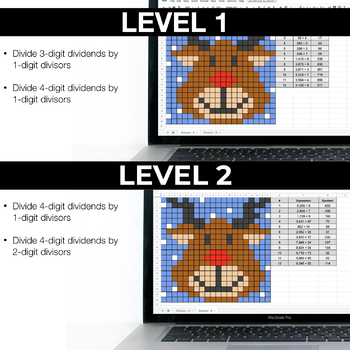Long Division - Digital Math Pixel Art - Mystery Picture Google Sheets5th, Homeschool
Subjects
Standards
Resource Type
Formats Included
• Zip
•Google Apps™
Pages
2 pagesThe Teacher-Author indicated this resource includes assets from Google Workspace (e.g. docs, slides, etc.).

Also included in

1. These self-checking Math Fluency Activities were made using Google Sheets. An Excel version is also included. There are 2 levels of each math activity, so you can differentiate as needed. ★ Included in this Bundle4th:> Comparing Fractions> Multi-Digit Multiplication5th:> Multiplying Decimal
\$9.00
\$12.00
Save \$3.00

Description

This self-checking Division Math Fluency Activity was made using Google Sheets. An Excel version is also included. There are 2 versions of the math activity, so you can differentiate as needed.

★ INCLUDED

Two pages are included in this resource.

Level 1: Divide 3-digit dividends by 1-digit divisors and Divide 4-digit dividends by 1-digit divisors

Level 2: Divide 4-digit dividends by 1-digit divisors and Divide 4-digit dividends by 2-digit divisors

*EXCEL VERSION

★ HOW IT WORKS

This math fluency activity is like a paperless color by code activity. Students enter their answers in the answer column. If the answers are correct, the image starts to appear. Colors do not appear for incorrect answers.

You can quickly assign each student a copy of the paperless math fluency activity using Google Classroom.

*Click Preview to View the Questions in Detail*

★ What you Need to Know

- This resource was created using Google Sheets

- You must have a Google email to use with Google Classroom

★ Simple Steps to Take AFTER Purchasing

1. Click on each link in the START HERE file

2. Create a copy of the Google Sheets

Standards & Learning Goals

5.NBT.6 - I can divide four-digit dividends by two-digit divisors. I can illustrate and explain the calculation by using equations, rectangular arrays, and/or area models.

Way to Use this Paperless Resource

- Morning Work

- Homework

- Computer Center

- Math Center

- Math Warm-Ups

PAPERLESS MATH CENTERS

More Digital Math Pixel Art

Total Pages
2 pages
Does not apply
Teaching Duration
N/A
Report this Resource to TpT
Reported resources will be reviewed by our team. Report this resource to let us know if this resource violates TpT’s content guidelines.

Standards

to see state-specific standards (only available in the US).
Find whole-number quotients of whole numbers with up to four-digit dividends and two-digit divisors, using strategies based on place value, the properties of operations, and/or the relationship between multiplication and division. Illustrate and explain the calculation by using equations, rectangular arrays, and/or area models.